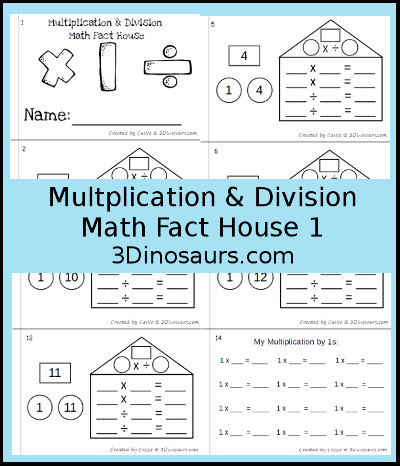# Multiplication & Division Math Fact BooksPlease print off as many or as few as you would like as many times as you would like. If you find any errors or typos please let me know. Download is below.

## Multiplication & Division Math Fact Number 1

Multiplication & Division Math Fact Number 1 contains 9 pages:

• Multiplication & Division Math Fact Number 1
• Singe Print Book
Click on the files below to download your copy of the Multiplication & Division Math Fact Number 1.
You can get the full set for \$4.00 at the links below.

Looking for additional ideas check out my Pinterest board.## Multiplication & Division Math Fact Books

Multiplication & Division Math Fact Books contains 316 pages:

• Math facts for Multiplication & Division from 1 to 12
• 12 Beginner Multiplication & Division Math Fact Books
• Single Print and Teacher Print Options for the Math Fact Books
• 15 pages for each begginner books
• 3 pages Types for the Review Multiplication & Division Math Fact Books
• Blank
• 1 number of the number bond filled out
• 2 numbers of the number bond filled out
• Option to use as a No-Prep Printable for the Review Multiplication & Division Math Fact Books
Click on the files below to download your copy of the Multiplication & Division Math Fact Books.
You can get the full set for \$4.00 at the links below.

Looking for additional ideas check out my Pinterest board.